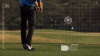# Newton’s First & Second Laws of Motion

From inertia to acceleration, USGA research engineer Jim Hubbell explains how Newton's Laws of Motion are demonstrated in every round of golf. "Science of Golf" is produced in partnership with the United States Golf Association and Chevron.

##### transcript

DAN HICKS reporting: Now you see it…now you don't! From zero to 175 miles per hour in a fraction of a second, today's top golfers can turn a golf ball into one of the fastest projectiles in sports.

SUZANN PETTERSEN (LPGA Tour Player): It's a nice feel when you can kind of crush it and it's straight down the middle, long and far.

HICKS: Suzann Pettersen is a professional golfer on the LPGA Tour, with top ten finishes in more than a dozen 2013 LPGA Tour events. In early 2014, Pettersen was second in the Women's World Golf Rankings.

PETTERSEN: You know there's nothing that beats hitting a pure golf shot, if it's a driver, if it's an iron or a wedge. It's a sweet feel and you know it.

HICKS: Whether crushing it or tapping it, getting the golf ball moving is explained by Newton's First and Second Laws of Motion.

JIM HUBBELL (United States Golf Association): Newton's laws can be seen in all aspects of the game of golf whether it's impact, whether it's the flight of the ball, or even the bounce and roll of the golf ball.

HICKS: Jim Hubbell is a research engineer at the United States Golf Association's Research and Test Center.

HUBBELL: Newton's first law basically states that a body at rest tends to stay at rest. A body in motion wants to stay in motion in a straight line unless it's affected by some external force.

HICKS: Newton's First Law of Motion, or the "Law of Inertia," says that objects tend to resist change in motion. This means the motion of an object with balanced or zero net forces will remain constant. The force of earth's gravity pulling down on a ball is balanced by the upward push of the tee. This balance of forces means there is a net, or total, force of zero - and the ball's state of motion remains the same. The force that a golf club applies to the ball is called unbalanced because there isn't an equal force to push back, or balance, the force of the club.

HUBBELL: So, a ball on a tee is going to stay on the tee unless there's some external force that changes that.

HICKS: An external force like a club. And just as force is needed to initiate motion, it's also needed to stop motion.

HUBBELL: The rolling ball would be an example of a ball rolling on a line or a constant direction at a uniform velocity is going to stay at that uniform velocity unless it's affected by some external force.

HICKS: Here, the external force is the friction of the grass. Newton's Second Law of Motion relates to the motion of an object when an unbalanced force causes a change in velocity. How quickly or slowly the object's velocity changes is called acceleration.

HUBBELL: Newton's second law really states or introduces the idea of acceleration. Acceleration of a body will be directly proportional to the force you apply to it and it will be inversely proportional to the mass of the object being accelerated.

HICKS: This means that if a large force is applied to a small mass, it results in a larger, or more rapid, acceleration. If the same force is applied to a larger mass, it results in a smaller, or slower, acceleration. This is represented by the equation acceleration equals force divided by mass - more commonly stated as F equals m times a.

HUBBELL: So it's pretty intuitive that a larger force will create a larger acceleration. A lighter body or a lighter-mass object will be more easily accelerated than a heavier object.

HICKS: At the USGA Test Center, Hubbell explains how they can measure the change in velocity of a golf ball at rest.

HUBBELL: This is our initial velocity measurement device. It measures, given a specific impact, how fast that ball will fly. Once the trigger is pulled, this striker comes out of the fly wheel while it's spinning and ultimately strikes the ball, and we'll measure the time it takes for the ball to fly to this far end of the test area. And that give us the initial velocity of the ball - a measurement of how fast the ball will come off the tee.

HICKS: The ball speed will be about 1.5 times the velocity of the club coming into impact.

HUBBELL: At a hundred-and-twenty-mile-an-hour clubhead speed, after the impact with the ball, the ball will take off at about a hundred and seventy-six miles per hour. So that's a pretty significant acceleration - from zero to a hundred-seventy-six miles per hour in five hundred microseconds.

HICKS: How much force accelerates the ball that quickly? Say the ball takes off at 176 miles per hour, or 78.7 meters per second. The collision happens in 500 microseconds. The change in velocity divided by collision time equals the acceleration on the ball. The mass of the ball is 1.62 ounces or .0459 kilograms. The mass of the ball multiplied by the acceleration equals the force of the club on the ball. That's a force of more than three quarters of a ton!

From teeing up to tapping in, Newton's Laws of Motion are in play, with inertia, force, mass, and acceleration found in every stroke.